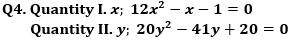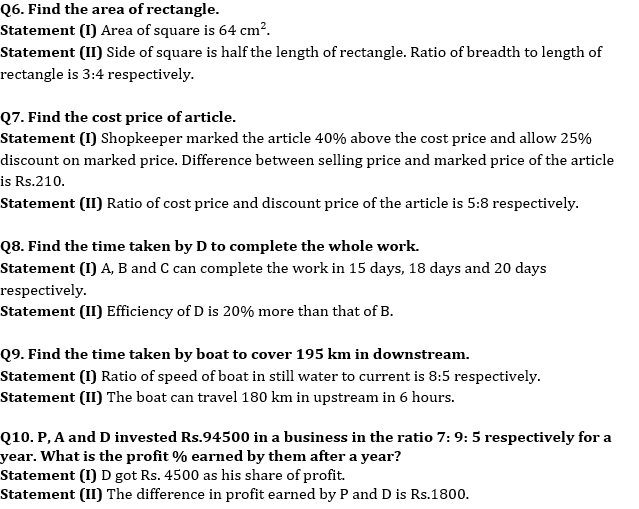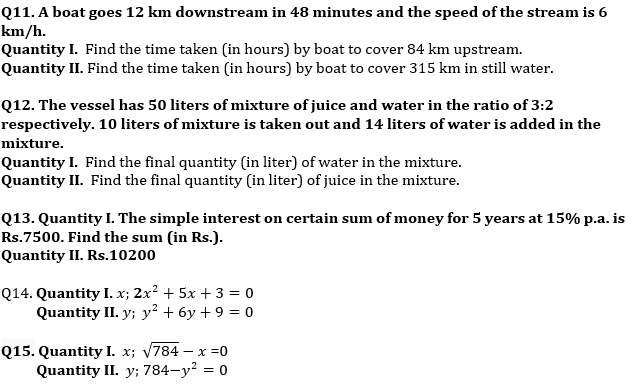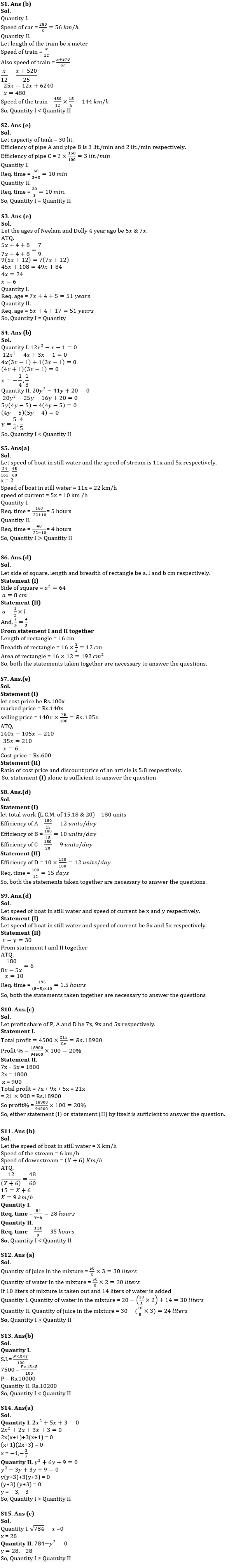Latest Banking jobs   »   Quantitative Aptitude Quiz For RBI Grade...

# Quantitative Aptitude Quiz For RBI Grade B Phase 1 2023 -03rd May

Directions (1–5): In the given questions, two quantities are given, one as ‘Quantity I’ and another as ‘Quantity II’. You have to determine relationship between two quantities and choose the appropriate option
(a) Quantity I > Quantity II
(b) Quantity I < Quantity II
(c) Quantity I ≥ Quantity II
(d) Quantity I ≤ Quantity II
(e) Quantity I = Quantity II or no relation

Q1. Quantity I. A car can travel 280 km in 5 hours. Find the speed of car. (in kmph)
Quantity II. A train can cross a pole and bridge having a length of 520 m in 12 secs and 25 secs respectively. Find the speed of the train (in kmph).

Q2. Pipe A and pipe B can fill the tank in 10 min and 15 min respectively. Efficiency of pipe C is 50% more than that of B.
Quantity I. Find the time taken (in min) by pipe C and A when both opened simultaneously to fill double the capacity of the tank.
Quantity II. Find the time taken (in min) by pipe C to fill the tank alone.

Q3. 4 years ago, the ratio of the ages of Neelam and Dolly is 5: 7. Eight years hence the ratio of their ages will be 7:9
Quantity I. Find the age of Dolly 5 years hence.
Quantity I. Find the age of Neelam 17 years hence.Q5. The ratio of speed of boat in still water to the speed of stream is 11: 5. A boat goes 24 km in 45 minutes downstream.
Quantity I. Find the time taken (in min) by boat to cover the distance of 160 km downstream?
Quantity II. Find the time taken (in min) by boat to cover the distance of 48 km upstream?

Directions (6-10): The following questions are accompanied by two statements i.e. statement (I) and statement (II). You have to determine which statements(s) is/are sufficient/necessary to answer the questions.
(a) Neither statement (I) nor statement (II) by itself is sufficient to answer the question.
(b) Statement (II) alone is sufficient to answer the question but statement (I) alone is not sufficient to answer the question.
(c) Either statement (I) or statement (II) by itself is sufficient to answer the question.
(d) Both the statements taken together are necessary to answer the questions, but neither of the statements alone is sufficient to answer the question.
(e) Statement (I) alone is sufficient to answer the question but statement (II) alone is not sufficient to answer the questionsDirections (11–15): In the given questions, two quantities are given, one as ‘Quantity I’ and another as ‘Quantity II’. You have to determine relationship between two quantities and choose the appropriate option
(a) Quantity I > Quantity II
(b) Quantity I < Quantity II
(c) Quantity I ≥ Quantity II
(d) Quantity I ≤ Quantity II
(e) Quantity I = Quantity II or no relationSolutions## FAQs

### How many sections are there in the RBI Grade B Phase 1 Exam?

There are 4 sections in the RBI Grade B Phase 1 Exam i.e. English Language, General Awareness, Quantitative Aptitude & Reasoning.

#### Congratulations!Union Budget 2023-24: Free PDF